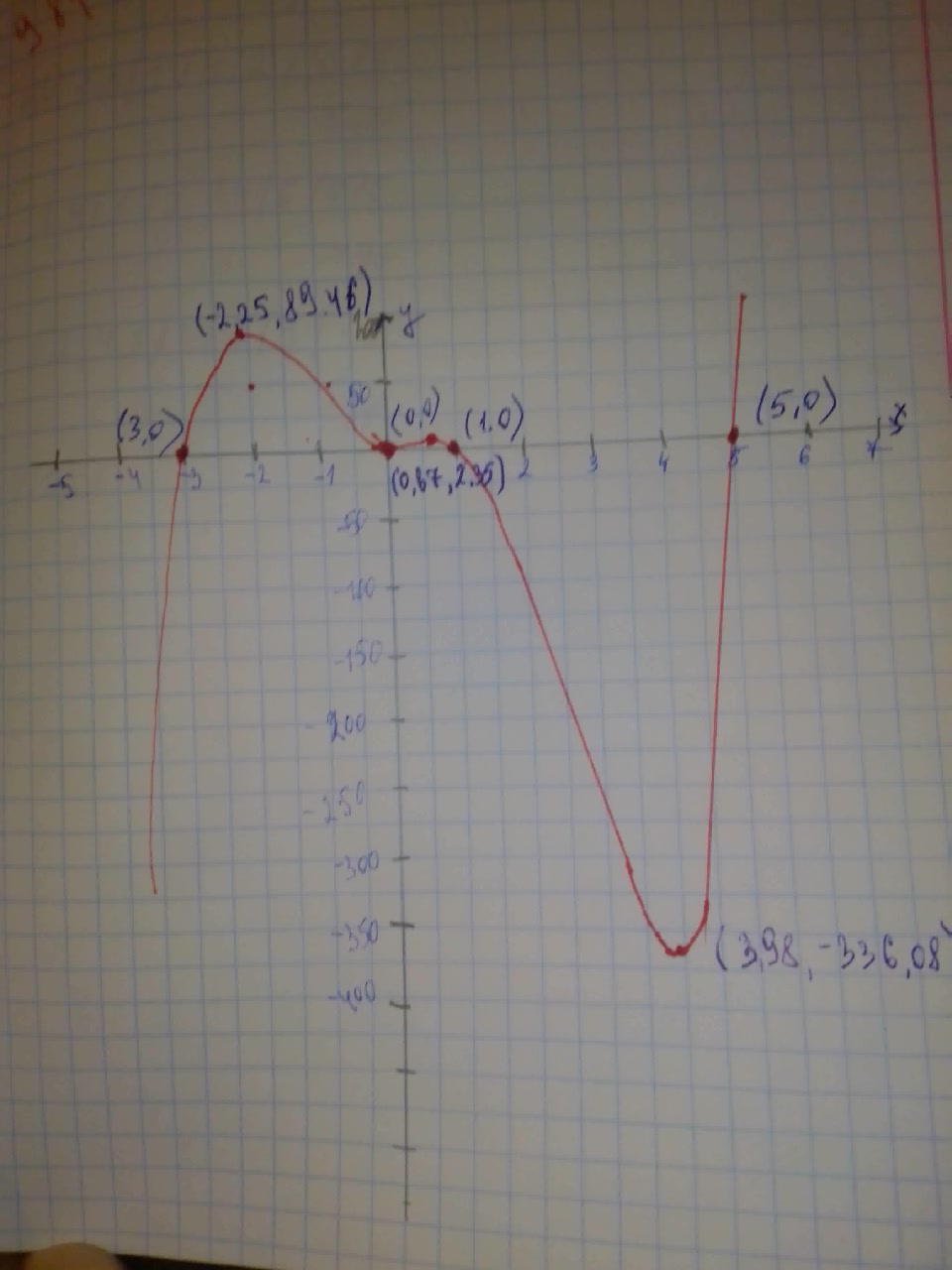# Graph each polynomial function. Factor first if the expression is not in factored form. f(x)=x^{2}(x-5)(x+3)(x-1)sjeikdom0 2021-02-12 Answered
Graph each polynomial function. Factor first if the expression is not in factored form. $f\left(x\right)={x}^{2}\left(x-5\right)\left(x+3\right)\left(x-1\right)$
You can still ask an expert for help

• Questions are typically answered in as fast as 30 minutes

Solve your problem for the price of one coffee

• Math expert for every subject
• Pay only if we can solve itPohanginah

$f\left(x\right)={x}^{2}\left(x-5\right)\left(x+3\right)\left(x-1\right)$ The function in factored form The function has four zeros 0,5,-3 and 1. So, the graph of f(x) crossed the x-axis at (0,0), (5,0),(-3,0), and (1,0) To find the y-intercept, substitute 0 for x in f(x) $f\left(x\right)={x}^{2}\left(x-5\right)\left(x+3\right)\left(x-1\right)$
$f\left(0\right)={0}^{2}\left(0-5\right)\left(0+3\right)\left(0-1\right)$ Substitute 0 for x
$=0$ So, the function f(x) crosses the y-axis at (0,0) Step 2 ${x}^{2}\cdot x\cdot x\cdot x={x}^{5}$ The leading coefficient is 1 Since the leading coefficient is positive and the function f(x) of degree 5 (odd degree) So, the end behavior is $x\to \mathrm{\infty },f\left(x\right)\to \mathrm{\infty }$
$x\to -\mathrm{\infty },f\left(x\right)\to -\mathrm{\infty }$

See the graph below